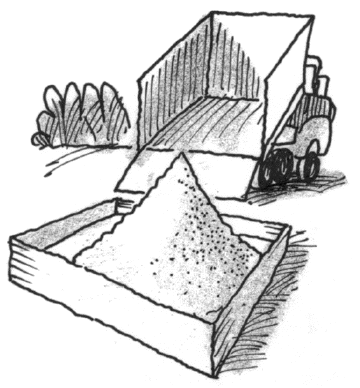### Home > CALC > Chapter 7 > Lesson 7.1.1 > Problem7-9

7-9.

Sand is being poured into a sandbox, making a conical pile with radius $r$ and volume $V$. Explain what $\frac { d V } { d t }$ and $\frac { d r } { d V }$ represent.

Remember that a derivative represents a slope, or rate of change.